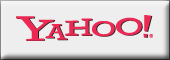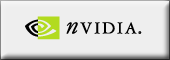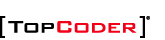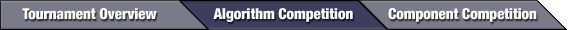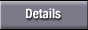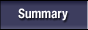mathijs is the new Algorithm Champion

See more photos!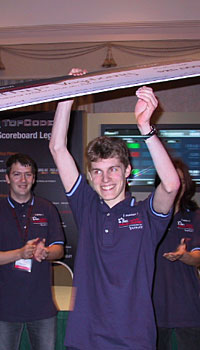\$20,000 and beating tomek, very sweet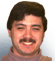by vorthys,
TopCoder Member
Friday, March 11, 2005
introduction by lbackstrom

The final round proved to be rather sparse on submissions. mathijs submitted the easy first, after only 9 minutes. tomek was a little bit slower, but he finished it in 15 minutes. Both coders moved on to the medium problem, while ploh and misof struggled with the easy problem. Eventually everyone but tomek gave up and moved on to the next problem. tomek quickly solved the medium problem for all the examples, but his code was too slow on a harder case. He would spend the rest of the coding phase trying to make it fast enough. In the meantime, the other three coders each opened both of the other two problems, but none of them made significant progress. As the time wound down, misof returned to the easy problem. When the coding phased ended, no one had submitted anything but the easy problem, and mathijs was in the lead. However, tomek less than 25 points behind, and so he had two attempts to get a successful challenge. Unforunately for him, both of his attempts failed, and he went into the systest in second place. As system tests rolled out, misof failed, while the other two passed. Congratulations to mathijs who continued the streak of upsets in this TCCC and finally unseated three-time tournament winner tomek.

Consider a recursive function count(n,context) that counts the number of strings of length n that can be legally placed after a string context. This function can be written as

```   function count(n,context) is
if n == 0 then return 1
else return count(n-1,context+'A') +
count(n-1,context+'B') +
count(n-1,context+'C')
```
Then the number of legal strings of length n is simply count(n,"").

However, this algorithm will be much too slow for even moderately large n, so we turn to either dynamic programming or memoization. But to use either of those techniques effectively, we need shared subproblems, which we obtain by trimming the context to include only that portion that can possibly affect upcoming strings. In particular, we replace the context with the longest suffix of the context that is also a prefix of one of the forbidden substrings. For example, if the context is "CBAABC" and the two forbidden substrings are "CAC" and "ABCB", then the only part of the context that is relevant is the trailing "ABC".

It is easy to add such a trimming step to an algorithm based on memoization. To use dynamic programming instead, you would enumerate over all possible prefixes of the two forbidden substrings.

## BasicSorting

Another memoization problem. This time you record what is known about the ordering of the files in some kind of state data structure (possibly a bit mask). For each pair of files, the data structure keeps track of whether one is known to be less than the other. Initially, nothing is known.

At each step, you try comparing all pairs of files whose relationship is not yet known, and return the best cost. The cost resulting from a particular comparison is not only the cost of the comparison itself, but also the cost of future comparisons (which we find recursively). There are two possible “futures” depending on which file was less than the other. We try both and keep the maximum cost. Then we return the minimum of these maximums, over all possible comparisons.

The tricky part is extending the current state with the result of a comparison. Say that we just discovered that file B is less than file C. By transitivity, we infer that B is less than D for all D known to be greater than C in the current state. Similarly, we infer that A is less than C for all A known to be less than B in the current state. Finally, we complete the transitive closure by inferring that A is less than D for all pairs A and D where A is known to be less than B and D is known to be greater than C.

Memoization and dynamic programming are usually interchangeable, so it is interesting to consider why memoization is preferable to dynamic programming for this problem. Dynamic programming depends on being able to enumerate the possible states, but for this problem, enumerating the states is not at all straightforward, at least if we want to do so efficiently. We don't actually want all the states, only those that are neither inconsistent (for example, states that claim A<B, B<C, and C<A) nor incomplete (for example, states that claim A<B and B<C but that claim no relationship between A and C). Using memoization, it is easy to avoid such states by immediately taking the transitive closure of each new state and by never comparing two files whose relationship is already known.

## LocalMax

Dang, that Fibonacci guy was really onto something. His numbers pop up in the strangest places...

Start by sorting the data points by their x-values. Then find all occurrences of three consecutive data points where the y-value of the middle data point is higher than the y-values of the other two data points. For each such occurrence, calculate the best result you could get by making your queries in this range, and return the best overall result.

Calculating the best result for a given range is almost embarrassingly simple. Consider the three x-values, and take the differences between the high and middle values and between the middle and low values. Let A be the smaller difference and let B be the larger difference. If N is 0, then the result is A+B, but if N > 0, then the result is Max(A/Fib(N-1), B/Fib(N)), where Fib(0) = Fib(1) = 1 and Fib(N) = Fib(N-1) + Fib(N-2).

Now, why does this work? Consider the base case, when N = 1. Then Max(A/Fib(N-1), B/Fib(N)) is just Max(A/1,B/1) = B. We can achieve this result by making a query infinitesimally on the B side of the middle x-value. If the corresponding y-value is high, then we know a local maximum is in the B range. If the corresponding y-value is low, then we know that a local maximum is in the A range (extended infinitesimally by our query). The worst case result is for it to fall in the B range.

For N > 1, we make a query by dividing the B range into two segments in the proportion of Fib(N-1) to Fib(N-2). If the y-value returned by the query is high, then we know a local maximum is in the B range. We continue with A' = B*Fib(N-2)/(Fib(N-1)+Fib(N-2)) = B*Fib(N-2)/Fib(N) and B' = B*Fib(N-1)/Fib(N). The remaining N-1 queries will yield a result of Max(A'/Fib(N-2),B'/Fib(N-1)) = Max(B/Fib(N),B/Fib(N)) = B/Fib(N).

On the other hand, if the y-value returned by the query is low, then we know the local maximum is either in the A range or in the Fib(N-2) segment of the B range. The worst case happens when A is larger than B*Fib(N-2)/Fib(N). Then the remaining N-1 queries will yield a result of Max((B*Fib(N-2)/Fib(N))/Fib(N-2), A/Fib(N-1)) = Max(B/Fib(N), A/Fib(N-1)).

It is interesting to compare the Fibonacci search strategy suggested by this problem with the "ternary" search algorithm familiar to many TopCoders (seen recently in some solutions to Disaster and Driving). The Fibonacci search converges substantially faster for the same number of queries.